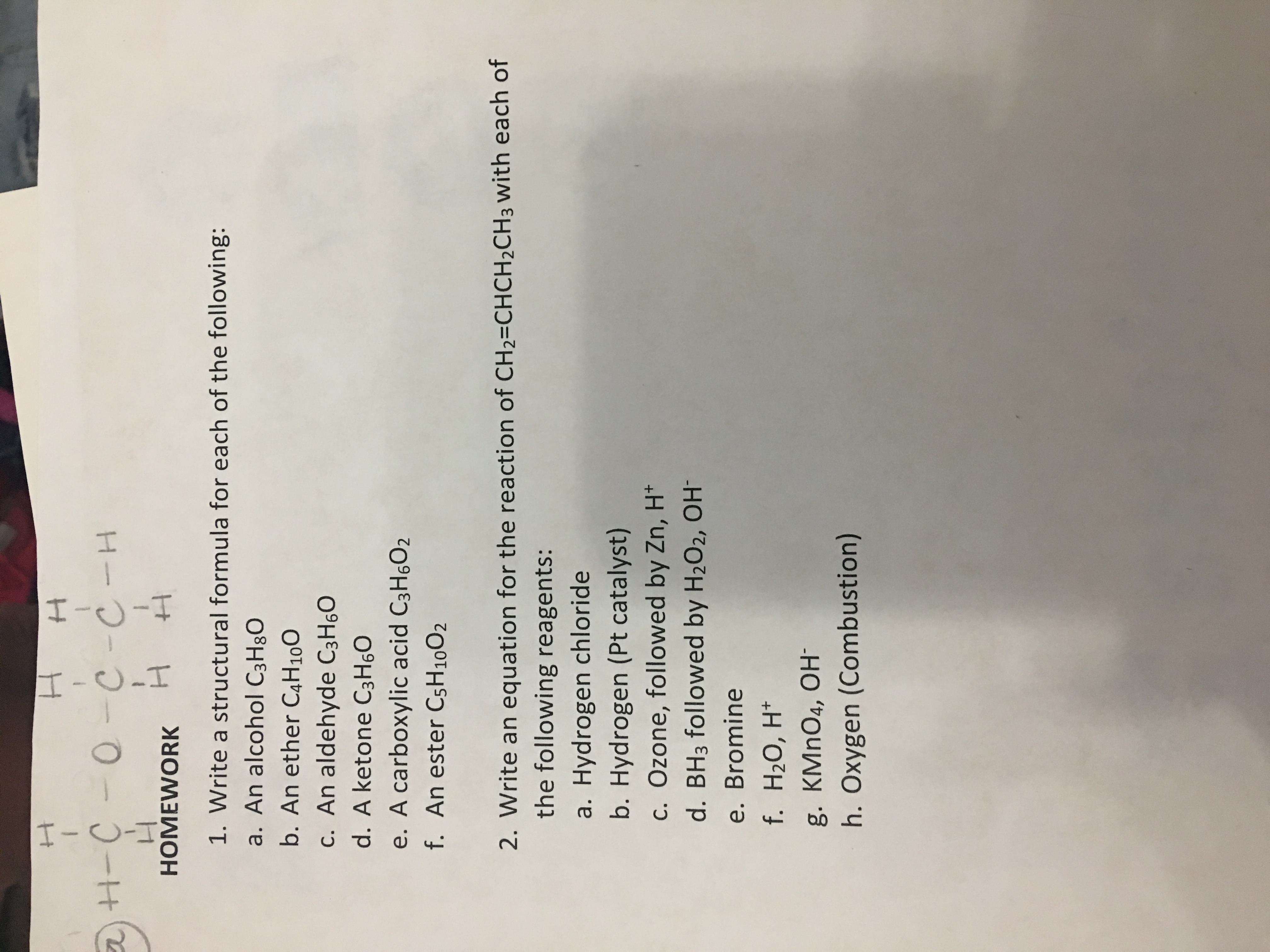# H H-C-OTC -C- C-CH n HOMEWORK 1. Write a structural formula for each of the following: a. An alcohol C3H80 b. An ether C4H100 c. An aldehyde C3H60 d. A ketone C3H60 e. A carboxylic acid C3H6O2 f. An ester CsH 10O2 2. Write an equation for the reaction of CH2=CHCH2CH3 with each of the following reagents: a. Hydrogen chloride b. Hydrogen (Pt catalyst) c. Ozone, followed by Zn, H* d. BH3 followed by H2O2, OH e. Bromine f. H2О, H* g. KMnO4, OH h. Oxygen (Combustion)

Questionhelp_outlineImage TranscriptioncloseH H-C-OTC -C- C-CH n HOMEWORK 1. Write a structural formula for each of the following: a. An alcohol C3H80 b. An ether C4H100 c. An aldehyde C3H60 d. A ketone C3H60 e. A carboxylic acid C3H6O2 f. An ester CsH 10O2 2. Write an equation for the reaction of CH2=CHCH2CH3 with each of the following reagents: a. Hydrogen chloride b. Hydrogen (Pt catalyst) c. Ozone, followed by Zn, H* d. BH3 followed by H2O2, OH e. Bromine f. H2О, H* g. KMnO4, OH h. Oxygen (Combustion) fullscreen

### Want to see the step-by-step answer?